• 利润预测值
千次阅读
2019-11-21 19:39:57

### 往期回顾：

在一元回归分析中，如果变量 y 与自变量 x的关系为非线性的，但又找不到适当的函数曲线来拟合，则可以采用一元多项式回归。

## 1. PolynomialFeatures

多项式生成函数：

sklearn.preprocessing.PolynomialFeatures(degree=2, interaction_only=False, include_bias=True)

参数说明：
degree：多项式阶数，默认值是2
interaction_only：值如果是True，则会产生相互影响的特征集
include_bias：是否包含偏差列

PolynomialFeatures 类通过实例化一个多项式，建立等差数列矩阵，然后进行训练和预测，最后绘制相关的图形。

from sklearn.linear_model import LinearRegression
from sklearn.preprocessing import PolynomialFeatures
import numpy as np
import matplotlib.pyplot as plt

# x表示企业成本，y表示企业利润
X = [, , , , , , , , , , , , , , ]
Y = [, , , , , , , , , , , , , , ]

# ================================ 线性回归分析 ================================
# 回归训练
clf = LinearRegression()
clf.fit(X, Y)

# 预测结果
X2 = [, , ]
Y2 = clf.predict(X2)
print(Y2)

res = clf.predict(np.array().reshape(-1, 1))
print(u'预测成本1200元的利润：%.1f' % res)

plt.plot(X, Y, 'ks')      # 绘制训练数据的散点图
plt.plot(X2, Y2, 'g-')    # 绘制预测数据的直线

# ================================ 多项式回归分析 ================================

xx = np.linspace(350, 950, 100)     # 350~950的等差数列

# 把训练好X值的多项式特征实例应用到一系列点上，形成矩阵
label="$y=ax^2+bx+c$", linewidth=2)
plt.legend()
plt.show()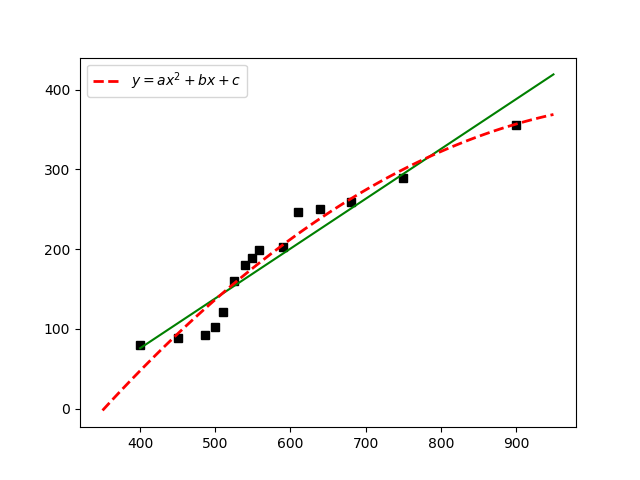这里使用R方(R-Squared) 来评价多项式回归预测的效果。R方也叫做确定系数(Coefficient of Determination), 表示模型对现实数据拟合的程度。

print('1 r - squared', clf.score(X, Y))


结果为：
1 r - squared 0.9118311887769117
2 r - squared 0.9407359949855933

### 文学模块：

九七五年二三月间，一个平平常常的日子，细的雨丝夹着一星半点的雪花，正纷纷淋淋地向大地飘洒着。时令己快到凉蛰，雪当然再不会存留，往往还没等落地，就已经消失得无踪无影了。黄土高原严寒而漫长的冬天看来不要过去，但那真正温暖的春天还远远地没有到来。
《平凡的世界》

更多相关内容
• 预测连续（实）... 代码在Octave版本4.2.1上成功执行 线性回归 在这个项目中，我实现了线性回归，并看到它在数据上的工作原理。 要开始使用该代码，您将需要下载代码并将其内容解压缩到您希望运行该代码的目录中...
• 文章目录吴恩达机器学习作业一：利用线性回归模型+梯度下降算法实现餐车利润预测（python实现）任务数据读取绘制数据,看下数据分布情况初始化的设置代价函数计算运行梯度下降算法图形绘制完整代码 任务 In this pa

## 吴恩达机器学习作业一：利用线性回归模型+梯度下降算法实现餐车利润预测（python实现）

该文是针对吴恩达机器学习作业任务一中，利用单变量线性回归模型实现餐车利润预测，对于任务二利用多元线线性回归模型实现房价预测见博客：传送门
当然，本文中的代价函数计算，梯度下降算法的实现也适用于多元线性回归。

### 任务

In this part of this exercise, you will implement linear regression with one
variable to predict profits for a food truck. Suppose you are the CEO of a
restaurant franchise and are considering different cities for opening a new
outlet. The chain already has trucks in various cities and you have data for
profits and populations from the cities.

利用线性回归模型预测餐车的利润：现在有一组数据，每个城市的人口以及在该城市餐车获得的利润，根据已有的这些数据，当给出人口数时预测餐车的利润。给出的数据第一列是一个城市的人口，第二列是一个城市的快餐车的利润。利润为负数表示亏损。

import numpy as np
import pandas as pd
import matplotlib.pyplot as plt


#### 数据读取

#读取数据
print(data)#### 绘制数据,看下数据分布情况

#绘制数据,看下数据分布情况
x=data['population']
y=data['profit']
plt.scatter(x,y)
plt.plot()
plt.xlabel('population')
plt.ylabel('profit')
plt.show()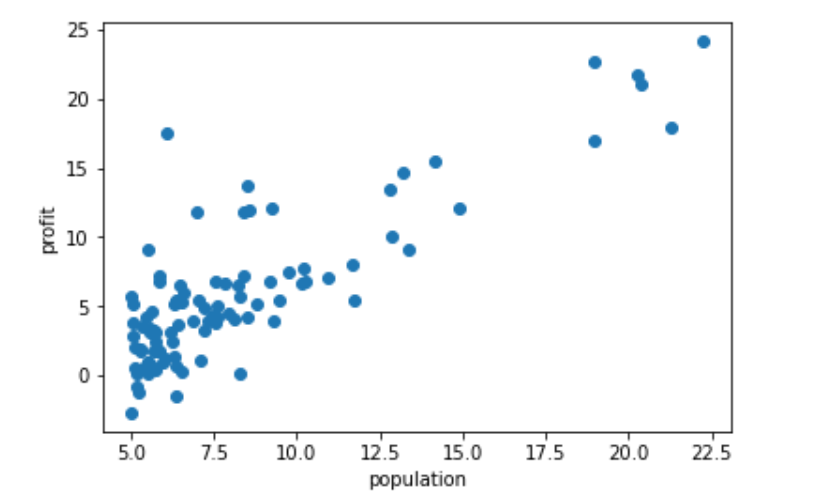#### 初始化值的设置

对于x0的值都为1，因此需要在训练数据的第一列添加一列，值为1
学习率设定为0.01,theta初始化值设为0，迭代次数设为1000次，m表示总共有多少个输入行

#在线性回归模型中，x0=1,即训练数据应该添加一列，值为1
data.insert(0, 'ones',1)
#初始化值的设置
alpha=0.01
theta=np.array([[0,0]])
iters=1000
m=len(X)
print(theta)

# X表示（x0,x1,...,xn）;Y表示实际利润
col=data.shape
X=np.array(data.iloc[:,0:col-1])
#print(X)
Y=np.array(data.iloc[:,col-1:col])
#print(Y)
X.shape,Y.shape,theta.shape


#### 代价函数计算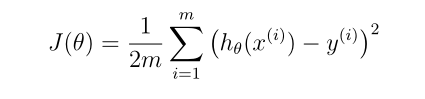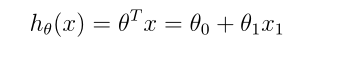def computeCost(X,Y,theta):
d=np.power(np.dot(X,(theta.T))-Y,2)
return np.sum(d)/(2*len(d))
computeCost(X,Y,theta) #当theta值为0时，代价为多少


32.072733877455676

#### 运行梯度下降算法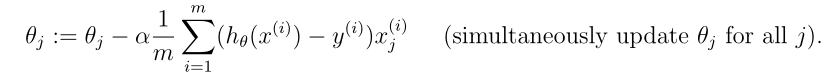在进行1000次迭代后，最新的theta值

def gradientDescent(X,Y,theta,iters,alpha):
for i in range(inters):
error=np.dot(X,(theta.T))-Y
temp=np.dot(error.T,X)
#print(temp)
theta=theta-alpha*temp/m #每一次迭代后，theta的值都会得到更新
#print(theta)
return theta

#得到第1000次迭代后的theta值，并计算此时的代价
print(theta)
cost=computeCost(X,Y,theta)
print(cost)


[[-3.78806857 1.18221277]]
4.4780276098799705

#### 图形绘制

#绘制线性回归模型，以及已有数据人口和利润的分布
def drawFigrue(data,theta):
fig,ax=plt.subplots(figsize=(12,8))
x1=data['population']
y1=data['profit']
ax.scatter(x1,y1,label='Training data')
x2=np.linspace(np.min(data['population']),np.max(data['population']),100)
y2=theta[0,0]+theta[0,1]*x2
ax.plot(x2,y2,'r',label='Prediction')
ax.legend(loc=2)
ax.set_xlabel('population')
ax.set_ylabel('profit')
ax.set_title("Predicted Profit vs. Population Size")
plt.show()

drawFigrue(data,theta)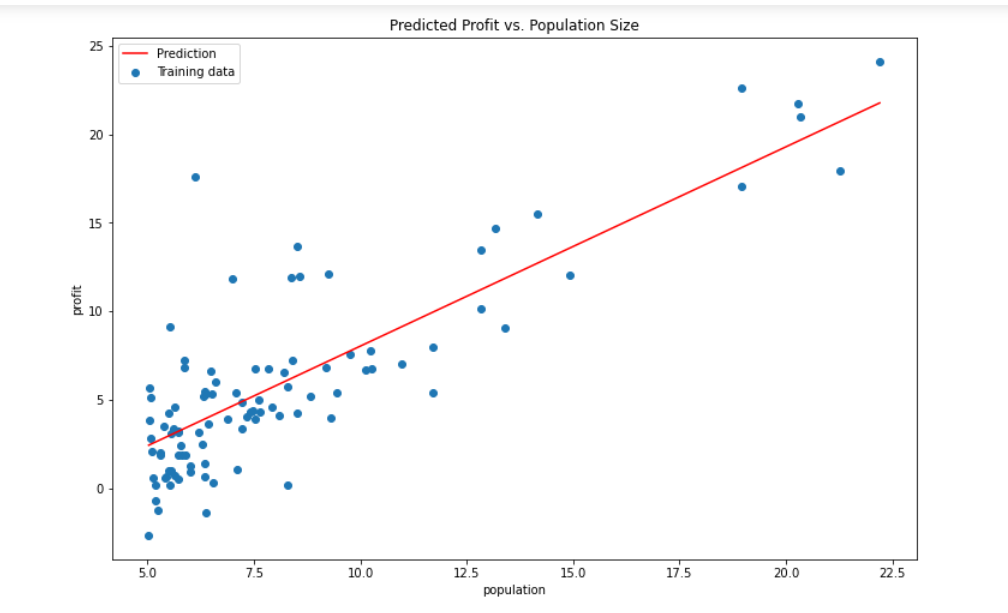#### 完整代码

import numpy as np
import pandas as pd
import matplotlib.pyplot as plt

#代价函数计算
def computeCost(X,Y,theta):
d=np.power(np.dot(X,(theta.T))-Y,2)
return np.sum(d)/(2*len(d))

#梯度下降算法，每次迭代和theta值的变化
for i in range(iters):
error=np.dot(X,(theta.T))-Y
temp=np.dot(error.T,X)
#print(temp)

theta=theta-alpha*temp/m #每一次迭代后，theta的值都会得到更新
#print(theta)
return theta

#绘制线性回归模型，以及已有数据人口和利润的分布
def drawFigrue(data,theta):
fig,ax=plt.subplots(figsize=(12,8))
x1=data['population']
y1=data['profit']
ax.scatter(x1,y1,label='Training data')
x2=np.linspace(np.min(data['population']),np.max(data['population']),100)
y2=theta[0,0]+theta[0,1]*x2
ax.plot(x2,y2,'r',label='Prediction')
ax.legend(loc=2)
ax.set_xlabel('population')
ax.set_ylabel('profit')
ax.set_title("Predicted Profit vs. Population Size")
plt.show()

#读取数据

#绘制数据,看下数据分布情况
x=data['population']
y=data['profit']
plt.scatter(x,y)
plt.plot()
plt.xlabel('population')
plt.ylabel('profit')
plt.show()

#在线性回归模型中，x0=1,即训练数据应该添加一列，值为1
data.insert(0, 'ones',1)
#初始化值的设置
alpha=0.01
theta=np.array([[0,0]])
iters=1000
m=len(X)
# X表示（x0,x1,...,xn）;Y表示实际利润
col=data.shape
X=np.array(data.iloc[:,0:col-1])
Y=np.array(data.iloc[:,col-1:col])

computeCost(X,Y,theta) #当theta值为0时，代价为多少

#得到第1000次迭代后的theta值，并计算此时的代价
print(theta)
cost=computeCost(X,Y,theta)
print(cost)
#绘制线性回归模型，以及已有数据人口和利润的分布
drawFigrue(data,theta)

展开全文python 机器学习 线性回归 梯度下降算法
• 下面来介绍一下在MATLAB中实现对某公司利润的灰色预测，及其与原始数据的比较： 1、首先在MATLAB的编辑器中写入下列代码： clear syms a b; c=[a b]'; A=[89677,99215,109655,120333,135823,159878,182321,209407...

下面来介绍一下在MATLAB中实现对某公司利润的灰色预测，及其与原始数据的比较：

1、首先在MATLAB的编辑器中写入下列代码：

clear
syms a b;
c=[a b]';
A=[89677,99215,109655,120333,135823,159878,182321,209407,246619,300670];
B=cumsum(A);  % 原始数据累加
n=length(A);
for i=1:(n-1)
C(i)=(B(i)+B(i+1))/2;  % 生成累加矩阵
end
% 计算待定参数的值
D=A;D(1)=[];
D=D';
E=[-C;ones(1,n-1)];
c=inv(E*E')*E*D;
c=c';
a=c(1);b=c(2);
% 预测后续数据
F=[];F(1)=A(1);
for i=2:(n+10)
F(i)=(A(1)-b/a)/exp(a*(i-1))+b/a ;
end
G=[];G(1)=A(1);
for i=2:(n+10)
G(i)=F(i)-F(i-1); %得到预测出来的数据
end
t1=1999:2008;
t2=1999:2018;
G
plot(t1,A,'o',t2,G)
title('原始数据与预测数据的比较')  %原始数据与预测数据的比较
xlabel('年份')
ylabel('利润')

2、命名保存至自定义路径下，点击运行，结果如下：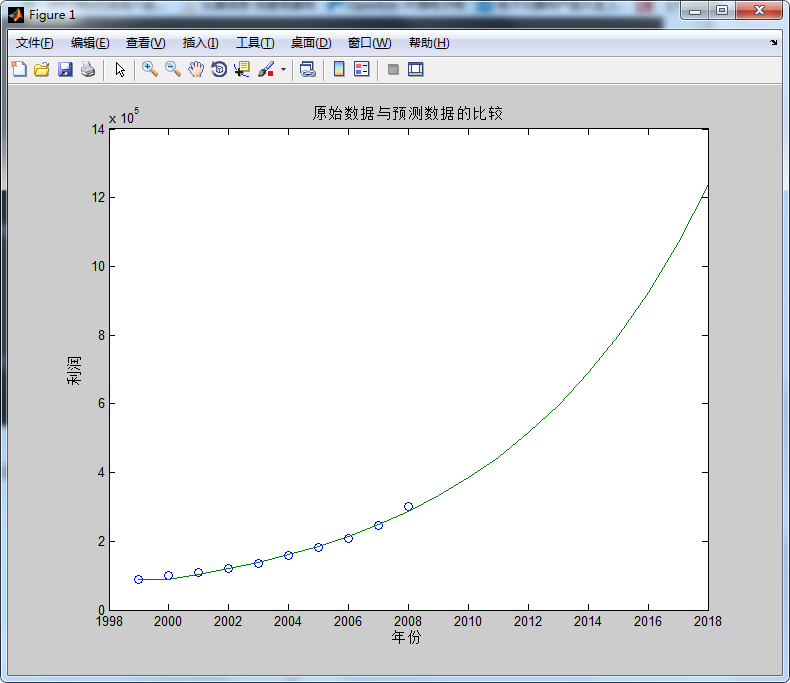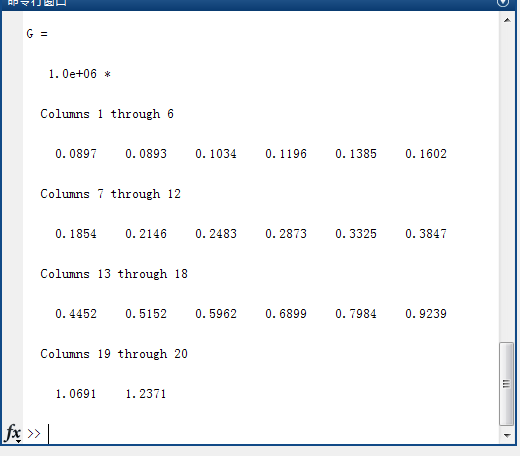看来该公司未来几年的趋势是比较可观的，至此基本介绍完毕，请大家继续关注！！！

展开全文MATLAB
• 这里需要引入残差的概念：残差，即预测值和真实值之间的差值。而方差齐性，指的就是满足随机分布的残差，如下图所示： 如何判断数据是否满足方差齐性呢？精确的判断是很难做到的，我们可以通过上图绘制X值与残差的...

往期推荐

机器学习100天学习计划 — 第1天 数据预处理

机器学习100天学习计划 — 第2天 线性回归

本文是机器学习100天学习计划的第三天，详细介绍了多元线性回归的原理和注意事项，并以预测企业利润为例进行实战演练。希望各位读者能耐心读下去。

何为多元线性回归？对比于前一天学习的线性回归，多元线性回归的特点是什么？

多元线性回归与简单线性回归一样，都是尝试通过使用一个方程式来适配数据，得出相应结果。不同的是，多元线性回归方程，适配的是两个及以上的特征（即X1、X2、...），而简单线性回归一般只有一个特征（X）。

另外，与简单线性回归相比，多元线性回归有一个显著的特点，即能拿到每个特征的权重，这样你能知道哪些因素对结果的影响最大。

多元线性回归特点和流程如下图所示：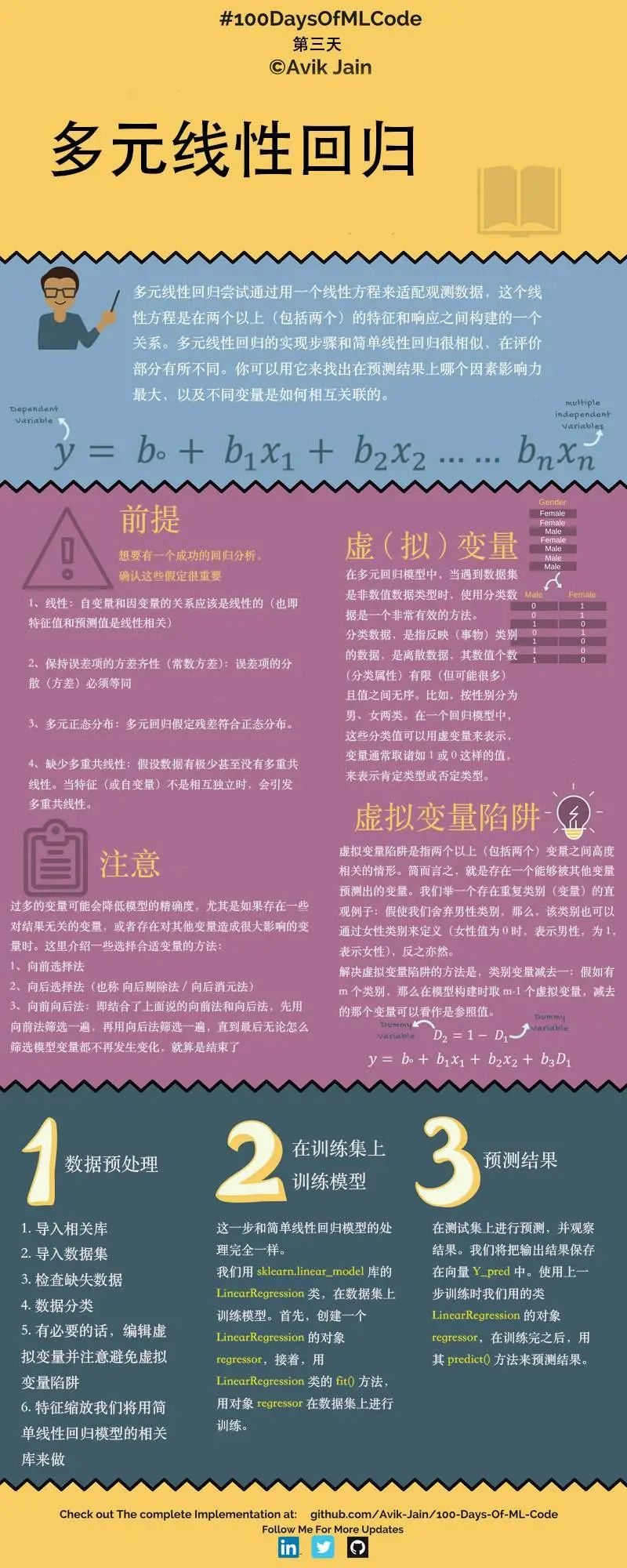请注意多元线性回归的以下4个前提：

1.线性：自变量和因变量的关系应该大致呈线性的。
2.呈现多元正态分布。
3.保持误差项的方差齐性（误差项方差必须等同）。
4.缺少多重共线性。

第一、二点比较好理解，第三、四点需要详细地讲一下：

第三点，保持误差项的方差齐性的意义: 首先，明确什么叫误差项？误差项也可以叫随机误差项，一般包括：

1)模型中省略的对被解释变量（Y）不重要的影响因素 (解释变量（X）)；
2)解释变量（X）和被解释变量（Y）的观测误差；
3)经济系统中无法控制、不易度量的随机因素。

再确定什么叫方差齐性：顾名思义，方差相等。与什么东西的方差呢？这里需要引入残差的概念：残差，即预测值和真实值之间的差值。而方差齐性，指的就是满足随机分布的残差，如下图所示：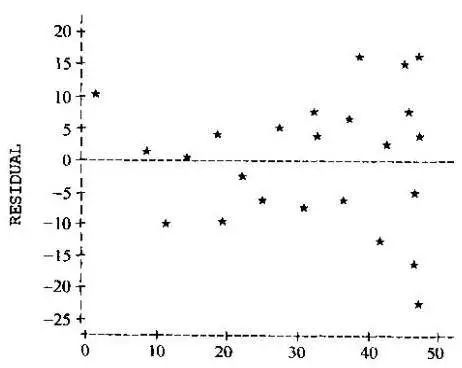如何判断数据是否满足方差齐性呢？精确的判断是很难做到的，我们可以通过上图绘制X值与残差的关系来大致估计，也可以用一个巧妙的方法：对残差做简单线性回归，如果得到的直线大致平行于X轴，则说明满足方差齐性

这种观察自变量与残差之间是否存在线性关系（BP法）或非线性关系（White检验）是较为常用的两种方差齐性检验的方法。

第四点，缺少多重共线性的意义：根据回归分析的结果，一般而言我们能发现自变量X1、X2、... 等因素对Y的影响。但是存在一种情况：如果各个自变量x之间有很强的线性关系，就无法固定其他变量，也就找不到x和y之间真实的关系了，这就叫做多重共线性。

有多种方法可以检测多重共线性，较常使用的是回归分析中的VIF值（方差膨胀因子），VIF值越大，多重共线性越严重。VIF怎么计算？VIF=1/(1-R^2), 其中R^2是样本可决系数。样本可决系数怎么计算？答案是通过残差，这里有详细的计算方法：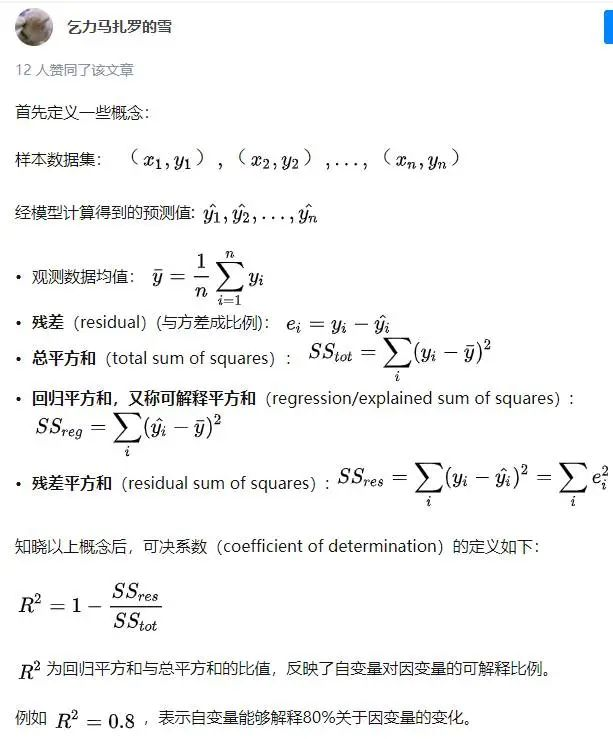一般认为VIF大于10时（严格是5），代表模型存在严重的共线性问题。

讲了这么多理论知识，大家可能觉得比较枯燥，但是实际上本文最难的也就是这些理论知识，希望大家能好好消化。下面正式开始实操部分。

## 0.准备

开始之前，你要确保Python和pip已经成功安装在电脑上，如果没有，请访问这篇文章：超详细Python安装指南 进行安装。

如果你用Python的目的是数据分析，可以直接安装Anaconda：Python数据分析与挖掘好帮手—Anaconda，它内置了Python和pip.

此外，推荐大家用VSCode编辑器，因为它有许多许多的优点：Python 编程的最好搭档—VSCode 详细指南

准备输入命令安装依赖，如果你没有VSCode编辑器，Windows环境下打开 Cmd (开始-运行-CMD)，苹果系统环境下请打开 Terminal (command+空格输入Terminal)，如果你用的是VSCode编辑器或Pycharm，可以直接在下方的Terminal中输入命令：

pip install pandas
pip install numpy
pip install matplotlib
pip install scikit-learn

## 本篇文章使用的50_Startups.csv文件，由研发开销、管理开销、市场开销、州和利润五列数据组成。

本文源代码和数据文件，可以关注Python实用宝典公众号，后台回复：机器学习3 下载。

## 1.数据预处理

导入库

import pandas as pd
import numpy as np

导入数据集

最后一列利润为Y值，其他均为X值。

dataset = pd.read_csv('50_Startups.csv')
X = dataset.iloc[:, :-1].values
Y = dataset.iloc[:, 4].values

将类别数据数字化

将“州”这一列数字化。

from sklearn.preprocessing import LabelEncoder, OneHotEncoder
labelencoder = LabelEncoder()
X[:, 3] = labelencoder.fit_transform(X[:, 3])
# 一共有3个州
onehotencoder = OneHotEncoder(categorical_features=)
X = onehotencoder.fit_transform(X).toarray()

躲避虚拟变量陷阱

原始数据如下：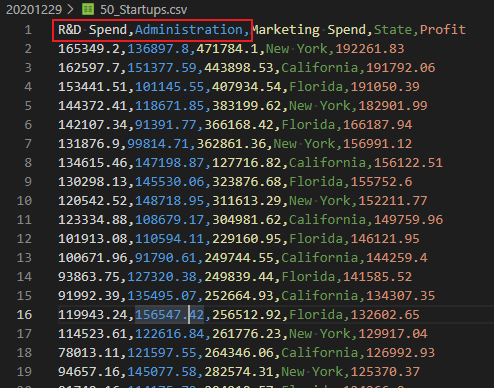在我们对“州”这列变量进行数字化后，会在前面出现三列one_hot变量，每一列代表一个州。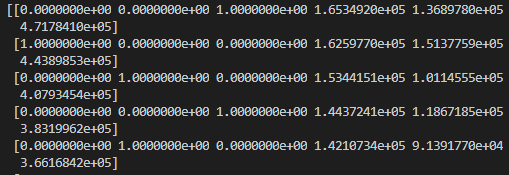这就出现虚拟变量陷阱了，比如第一列我们能通过另外两列的值得到：如果2、3列为0，第1列肯定为1，如果2、3列存在不为0的值，则第1列肯定为1。

因此，这里第一列没有使用的必要。

X = X[: , 1:]

拆分数据集为训练集和测试集

from sklearn.model_selection import train_test_split
X_train, X_test, Y_train, Y_test = train_test_split(X, Y, test_size = 0.2, random_state = 0)

## 第二步：训练模型

与简单线性回归一样，使用LinearRegression即可实现多元线性回归（sklearn已经帮你处理好多元的情况）。

from sklearn.linear_model import LinearRegression
regressor = LinearRegression()
regressor.fit(X_train, Y_train)

## 第三步：预测结果

一行搞定：

y_pred = regressor.predict(X_test)
print("real:", Y_test)
print("predict:", y_pred)

看看效果：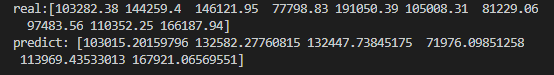画个图看看对比效果：

map_index = list(range(len(Y_test)))
plt.scatter(map_index, Y_test, color='red')
plt.plot(map_index, y_pred, color='blue')
plt.show()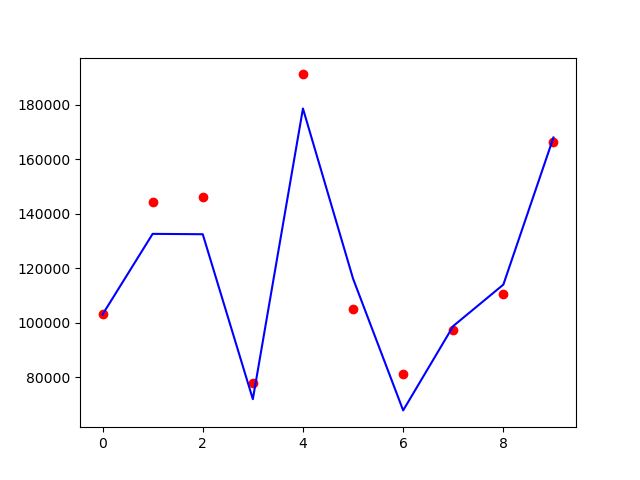蓝色的线是预测值，红色的点是真实的值，可以看到预测效果还是不错的。本文源代码和数据文件，可以关注Python实用宝典公众号，后台回复：机器学习3 下载。

我们的文章到此就结束啦，如果你喜欢今天的Python 实战教程，请持续关注Python实用宝典。

有任何问题，可以在公众号后台回复：加群，回答相应红字验证信息，进入互助群询问。

原创不易，希望你能在下面点个赞和在看支持我继续创作，谢谢！

点击下方阅读原文可获得更好的阅读体验

Python实用宝典 (pythondict.com)
不只是一个宝典
欢迎关注公众号：Python实用宝典展开全文python 机器学习 人工智能 编程语言 逻辑回归
• 为了制定满足风险要求的这一策略，从发电企业的角度出发，通过分析历史预测出清价的误差，统计其概率分布，最后得到误差在不同置信度下的VaR，并且通过VaR来指导发电企业进行段电量和段电价的申报。计算实例表明...
• 中低成本粘性组合,分析师盈余预测结果能证明现金流量持续性高于应计利润持续性,而高成本粘性组合,分析师盈余预测会高估应计利润持续性,盈余预测有效性较差。研究结果表明:只有低成本粘性组合能反映非操纵性应计利润...
• 预处理步骤：探索缺失； 中心尺度，和 boxcox 偏斜的数字预测变量； 为因子预测变量创建虚拟变量； 去除冗余的高度相关变量； 删除接近零的预测变量以形成缩减集，但也保留接近零以及完整的集合（可用于不同的...
• 主要为大家详细介绍了Tensorflow酸奶销量预测分析，具有一定的参考价值，感兴趣的小伙伴们可以参考一下
• 本次实验的涉及要素较多主要分为以上几个比较重要的... 接下来就是预测我们的结果： df_test["predict_revenue"] = xgb.predict(df_test[model_list]) df_test["predict_revenue"] 预测结果如上图所示，本次实验结束！sklearn 机器学习 python
• 具体代码请参照GitHub：https://github.com/RobertWang2/Python-/tree/master/ml-class-ex/machine-learning-ex1 本次是运用梯度下降法     红色的是数据集，蓝色的是线性...红色的位置 就是理想的参数  ...
• 实验过程： 导入需要用到的包 获取股票数据 寻找并构造预测因子 利用因子给股票池中的股票打分 检验收益率预测的准确性 阅读python编程规范 实验目标： 理解预测因子的作用 掌握使用python进行编程 熟练如何定义...
• 利润= 营收-产品成本 1计算期望营收和产品成本 1.1运行1000次公司营收模拟 需要数据： rev 期望营收 =170????????????????????????????（标准差170million（标准差20 million） 产品成本
• ## 常见的预测算法

千次阅读 2018-01-11 11:22:29
常见的预测算法 常见的预测算法有 1.简易平均法，包括几何平均法、算术平均法及加权平均法; 2.移动平均法，包括简单移动平均法和加权移动平均法; 3,指数平滑法，包括 一次指数平滑法和二次指数平滑法，三...算法
• 针对电信客户流失数据集存在的数据维度过高及单一分类器预测效果较弱的问题，结合过滤式和封装式特征选择方法的优点及组合分类器的较高预测能力，提出了一种基于Fisher比率与预测风险准则的分步特征选择方法结合组合...
• 使用RMSE在模型一步预测和实际之间分析了模型的性能。 表现最好的模型是Prophet，然后是三重指数平滑模型。 找到最佳绩效模型之后，我将其用于预测公司中各个商店的未来利润。 结果可以在sales_plots文件夹中...
• 时间序列预测 预测时间段by Kirill Dubovikov 通过基里尔·杜博维科夫(Kirill Dubovikov) 如何进行下一个时间序列预测项目 (How to rock your next time series forecasting project) Time series forecasting is ...大数据 编程语言 python 机器学习 人工智能
• 因此，有效地预测股票走势可以将投资风险最小化，利润最大化。 在我们的论文中，我们使用时间序列预测方法来预测和可视化预测。 我们的预测重点将基于使用历史数据和 ARIMA 模型的技术分析。 自回归综合移动平均 ...
• TensorFlow自自定定义义损损失失函函数数来来预预测测商商品...预测值比真实销量大商家损失的是生产商品的成本 而如果预测少了 预测 值比真实销量小损失的则是商品的利润因为一般商品的成本和商品的利润不会严格相等比
• 遵循多元回归方法，将估值倍数作为因变量，将价值驱动因素作为自变量，以基于470个公司观察获得预测倍数。 通过对基本变量的倍数进行回归，可以获得每个扇区的最适合倍数和倍数的关键驱动因素。 基于均方根误差...
• 波动率预测是当前金融工具中一个有趣的具有挑战性的话题，因为它与利润直接相关。 有许多与波动性直接相关的风险和回报。 因此，预测波动性成为金融领域最可有可无的话题。 GARCH 分布在风险度量和期权定价中起着...
• 文章目录前言一、CNN可以做预测吗？二、实现步骤1.准备工作（下载数据集）2.实战开始（1）导入所需包总结 前言 在大多数同学的眼中，CNN可能只是用来做图像识别的，没办法将他和预测放在一起，我也是，至少我几个...深度学习 python 机器学习 神经网络
• 在此基础上以供给侧结构性改革为前提,运用指数平滑法构建煤炭价格模型,从而求得煤炭前一年度月份价格平滑值,确定a值,并得到二次指数平滑法预测月份价格模型,根据每月时间点T的推移,得出相应月份煤炭价格预测值。...
• 预测与决策课件：第九讲 投入产出分析预测法-new.ppt
• 主要介绍了TensorFlow自定义损失函数——预测商品销售量,本文给大家介绍的非常详细，具有一定的参考借鉴价值，需要的朋友可以参考下
• 它还将在最终报告（按预测利润百分比排序的数据框）中过滤掉测试 RMSE 高于临界的任何股票，默认值为 0.05。 其他模式见下文。 LSTM 神经网络基于 Randerson112358 在 Adapted to Predict 24 小时开盘差异而不是......